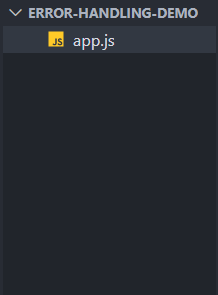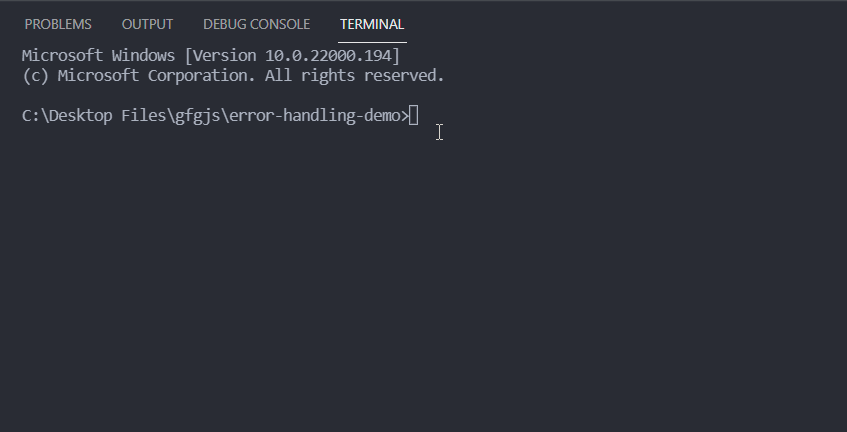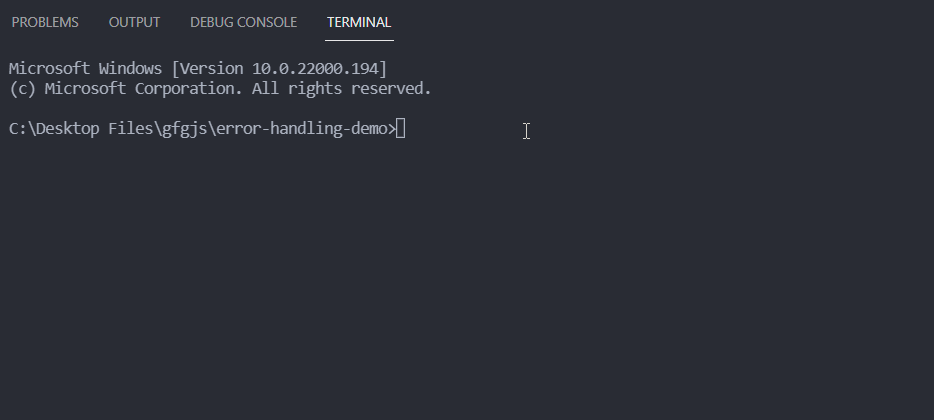# How to handle errors for async code in Node.js ?

• Last Updated : 09 Oct, 2021

Asynchronous operation in JavaScript is the operation that does not block further operations. It means that if we perform an asynchronous operation at a certain point in the code then the code after that is executed and does not wait for that asynchronous operation to be completed. An example of an asynchronous operation in Node.js is when we request some data from a web server.

If we want to handle the error for asynchronous code in Node.js then we can do it in the following two manners.

• Handle error using callback
• Handle Promise rejection

Handle error using callback: A callback function is to perform some operation after the function execution is completed. We can call our callback function after an asynchronous operation is completed. If there is some error we can call the callback function with that error otherwise we can call it with the error as null and the result of the asynchronous operation as the arguments.

Project Setup:

Step 1: Install Node.js if you haven’t already.

Step 2:  Create a folder for your project and cd (change directory) into it. Create a new file named app.js inside that folder.

Project Structure: After following the steps your project structure will look like the following.In the code example mentioned below, we have simulated an async operation using setTimeout() method. We perform a divide operation that returns the result of the division after 1 second and if the divisor is zero we pass an error Instance to the callback method. If there is no error, we call the callback function with the error as null and the result of division as the arguments. The error and result are handled inside our callback function.

## app.js

 `const divide = (a, b, callback) => { ` `  ``setTimeout(() => { ` `    ``if` `(b == 0) { ` `      ``callback(``new` `Error(``'Division by zero error'``)); ` `    ``} ``else` `{ ` `      ``callback(``null``, a / b); ` `    ``} ` `  ``}, 1000); ` `}; ` ` `  `divide(10, 2, (err, res) => { ` `  ``if` `(err) { ` `    ``console.log(err.message); ` `  ``} ``else` `{ ` `    ``console.log(`The result of division = \${res}`); ` `  ``} ` `}); ` ` `  `divide(5, 0, (err, res) => { ` `  ``if` `(err) { ` `    ``console.log(err.message); ` `  ``} ``else` `{ ` `    ``console.log(`The result of division = \${res}`); ` `  ``} ` `});`

Step to run the application: You can execute your app.js file using the following command on the command line.

`node app.js`

Output:Handle Promise rejection: Promise in Node.js is a way to handle asynchronous operations. Where we return a promise from an asynchronous function, it can later be consumed using then() method or async/await to get the final value. When we are using the then() method to consume the promise and we have to handle the promise rejections, then we can a catch() call to then() method call. Promise.catch() is a method that returns a promise and its job is to deal with rejected promise.

Syntax:

```// func is an async function
func().then(res => {
// code logic
}).catch(err => {
// promise rejection handling logic
})```

Now if we want to handle the Promise rejections using async/await then we can easily do it using a simple try/catch block as shown in the syntax given below.

```const hello = async () => {
try {
// func is an async function
const res = await func();
} catch(err) {
// error handling logic
}
}```

In the below example, we simulate an asynchronous function with setTimeout() method and perform the divide operation inside an async function that returns a Promise. If the divisor is zero, we reject the promise with an error otherwise we resolve it with the result of division.

## app.js

 `const divide = async (a, b) => { ` `  ``return` `new` `Promise((resolve, reject) => { ` `    ``setTimeout(() => { ` `      ``if` `(b == 0) { ` `        ``reject(``new` `Error(``'Division by zero error'``)); ` `      ``} ``else` `{ ` `        ``resolve(a / b); ` `      ``} ` `    ``}, 1000); ` `  ``}); ` `}; ` ` `  `// Consuming the promise using then() method ` `// and handling the rejected promise using ` `// catch() method ` `divide(5, 0) ` `  ``.then((res) => { ` `    ``console.log(`The result of division is \${res}`); ` `  ``}) ` `  ``.``catch``((err) => { ` `    ``console.log(err.message); ` `  ``}); ` ` `  `// This function is immedietly invoked after ` `// its execution. In this case we consume the ` `// promise returned by divide function() using ` `// async/await and handle the error using ` `// try/catch block ` `(async () => { ` `  ``try` `{ ` `    ``const res = await divide(10, 5); ` `    ``console.log(`The result of division is \${res}`); ` `  ``} ``catch` `(err) { ` `    ``console.log(err); ` `  ``} ` `})();`

Output:My Personal Notes arrow_drop_up
Recommended Articles
Page :